### CME 102 ― Ordinary Differential Equations for Engineers Star

My twin brother Afshine and I created this set of cheatsheets when I was a TA for Stanford's CME 102 class in Spring 2018. They can (hopefully!) be useful to all future students taking this course as well as to anyone else interested in learning the fundamentals of Ordinary Differential Equations.

#### Cheatsheet

First-order ODEs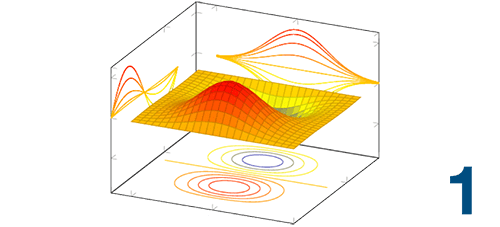• • Direction field method, separation of variables, equilibrium
• • Linear first-order technique, system of linear ODEs
• • Existence and uniqueness, initial value numerical methods
Second-order ODEs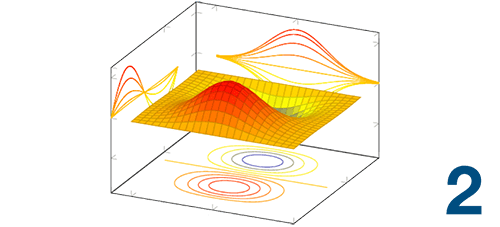• • General methods of resolution, standard form, linear dependency
• • Linear homogeneous: variable coefficients, constant coefficients
• • Linear inhomogeneous: variable coefficients, constant coefficients
Applications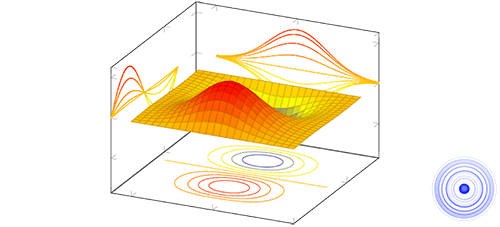• • Gravitational, spring, friction forces, Newton's second law
• • Spring-mass system: free and forced oscillation
• • Boundary value problems: direct and shooting methods

#### Mathematical tools

Linear Algebra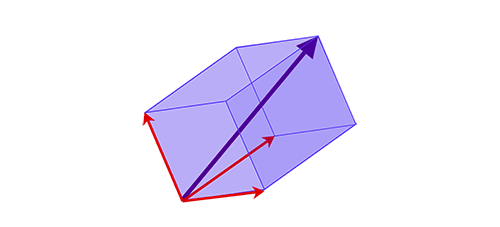• • Vector, matrix and general operations
• • Characteristic equation, determinant, eigenvector/eigenvalue
• • Partial fractions and main formulas
Calculus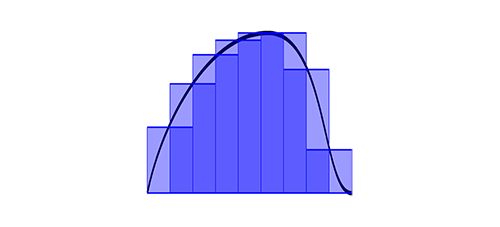• • Primitive function and formulas
• • Integration by parts
• • Laplace transform, common pairs and main operations
Trigonometry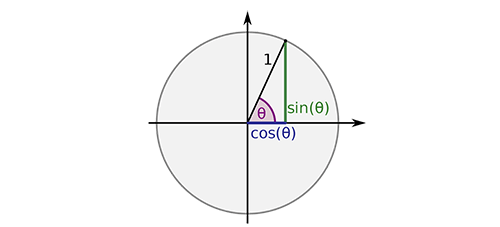• • Trigonometric functions and their inverse
• • Trigonometric identities
• • Euler's formula, Kashani theorem and law of sines

#### Matlab tips

Getting started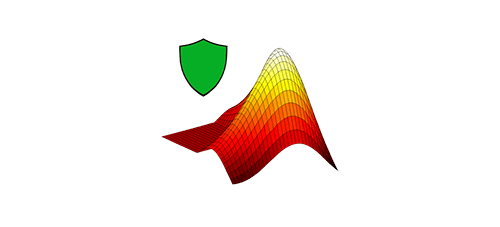• • Installation and first steps
• • Built-in functions, vectors, matrices, operators
• • Functions, plots and good practices